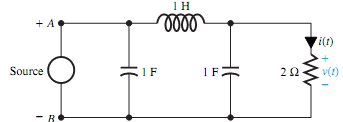## Find the current source, Electrical Engineering

Assignment Help:

Q. Consider the circuit shown in Figure.

(a) Given v(t) = 10e-t V, find the current source is(t) needed.

(b) Given i(t) = 10e-t A, find the voltage source v (t) needed.#### Delay line in CRO, why we use delay line in CRO what happens if we not use ...

why we use delay line in CRO what happens if we not use it?

#### Find the steady state values, Question: Consider the circuit shown in F...

Question: Consider the circuit shown in Figure. There is no initial energy stored in the circuit until time t=0 when the switch is closed. (i) Transform the given circ

#### Frequency response of your amplifier, a) What is meant by frequency respons...

a) What is meant by frequency response and how would you check the performance of your amplifier? b) Calculate the Voltage Gain of your amplifier at a range of frequencies suffic

#### Differences between enhancement and depletion mosfet, Q. What Are the Diffe...

Q. What Are the Differences Between Enhancement And Depletion Mosfet? Depletion MOSFET:Here a slab of p_type material is formed from a silicon base and referred to as substrate

Q. Show Balanced three-phase loads? Three-phase loads can be connected in either wye (also known as star or Y) or delta (otherwise known as mesh or ). If the load impedances i

#### How is segmentation implemented in typical microprocessors, How is segmenta...

How is segmentation implemented in typical microprocessors? The first advantage is as memory segmentation has is that only 16 bit registers are needed both to store segment bas

#### If a feeder circuit-breaker trips what action is required, Q. If a feeder c...

Q. If a feeder circuit-breaker trips, what action is needed? Ans: First isolate the faulty feeder check the faulty area on which fault the breaker was tripped (open circuit,

#### Electrical science value2 of 4, discuss the generator action in a dc motor...

discuss the generator action in a dc motor

#### Find the average power absorbed by each element, Q. Let v(t) = Vmax cos ωt ...

Q. Let v(t) = Vmax cos ωt be applied to (a) a pure resistor, (b) a pure capacitor (with zero initial capacitor voltage, and (c) a pure inductor (with zero initial inductor current)

#### Bronze is an alloy of which metal, Bronze is an alloy of  (A) Copper....

Bronze is an alloy of  (A) Copper.  (B)  Aluminium. (C)  Silver.  (D)  Carbon. Ans: Bronze is an alloy of copper.